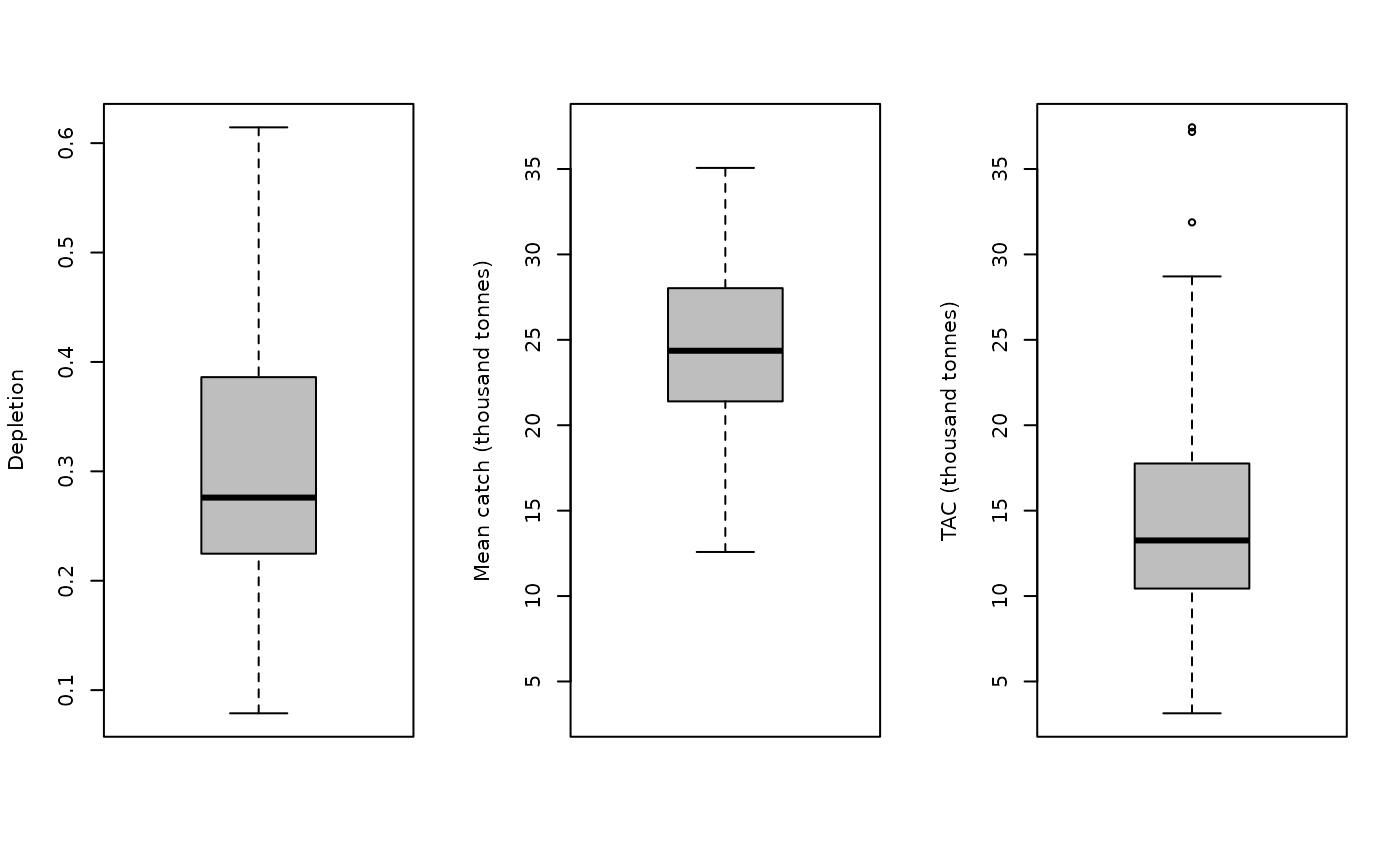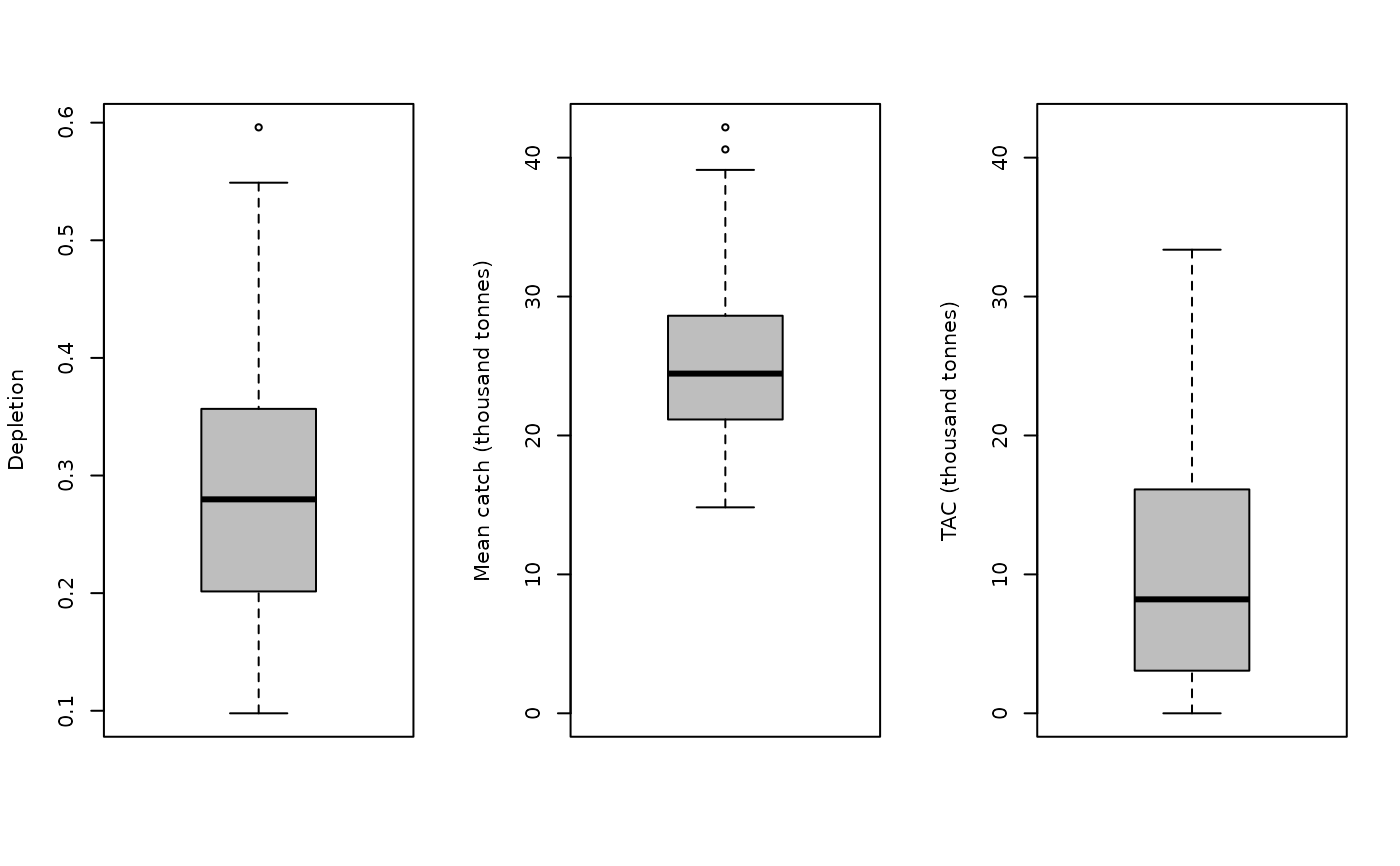A simple average catch-depletion MP that was included to demonstrate just how informative an estimate of current stock depletion can be.

MCD(x, Data, reps = 100, plot = FALSE)

MCD4010(x, Data, reps = 100, plot = FALSE)

## Arguments

x

A position in the data object

Data

A data object

reps

The number of stochastic samples of the MP recommendation(s)

plot

Logical. Show the plot?

## Value

An object of class Rec-class with the TAC slot populated with a numeric vector of length reps

## Details

The TAC is calculated as: $$\textrm{TAC} = 2 \bar{C} D$$ where $$\bar{C}$$ is mean historical catch, and $$D$$ is estimate of current depletion.

The TAC is modified by a harvest control rule in MCD4010.

## Functions

• MCD: The calculated TAC = 2 \* depletion \* AvC

• MCD4010: Linked to a 40-10 harvest control rule

## Required Data

See Data-class for information on the Data object

MCD: Cat, Dep

MCD4010: Cat, Dep

## Rendered Equations

See Online Documentation for correctly rendered equations

T. Carruthers

## Examples

MCD(1, MSEtool::Atlantic_mackerel, plot=TRUE)#> TAC (median)
#>     14.02198
MCD4010(1, MSEtool::Atlantic_mackerel, plot=TRUE)#> TAC (median)
#>     11.38775